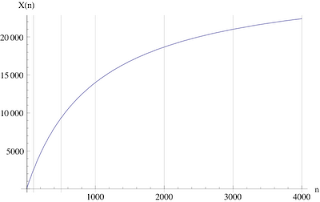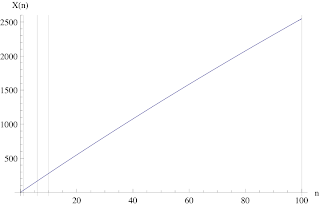## Thursday, April 9, 2009

### Assessing USL Scalability with Mixed Business Functions

Professional capacity planner, Raja C., has been applying my Universal Scalability Law (USL) in some fascinating and progressive ways. By that I mean, fascinating to me, because I hadn't thought about applying the USL model in the same way he has; I don't have a real job, you understand. On the other hand, this may well represent the situation that many of you are faced with on a day-to-day basis, so I'd like to present and discuss Raja's question here in some detail.

In a nutshell, whereas I typically present the USL model as having a natural fit with data collected from a controlled environment (e.g., a load testing rig), Raja has been pioneering the application of USL directly to production data. Production data presents potential problems for any kind of modeling because those data typically do not represent an environment in steady-state, i.e. they are likely to reflect significant transients. With that caveat in mind, it is sometimes just a matter of finding periods where steady-state conditions are well approximated. One such period could be a peak time when traffic is maximized. As long as nothing pathelogical is happening (i.e., huge swings in throughput or response times), we may take the peak period as being near steady state. As well, it should be possible to find other off-peak periods that also approximate steady state. In other words, viewed across an entire day, a production environment probably cannot be regarded as conforming to the definition of steady-state, but during the day, there will often be windows of time where steady-state conditions are well approximated.

Even if we can declare the peak-period to be a steady-state period on a large-scale production system, the USL is not defined for different types of users doing different kinds of work, i.e., mixed workloads. The USL, being a relatively simple model, carries no notion of heterogeneous workloads. For that, you would normally resort to a more sophisticated modeling tool, like PDQ, for example. Another challenge is, how do we determine the throughput X(1) for a single user (N = 1), in order to be able to do the required normalization for the relative capacity function in USL calculations? In particular, what does a "single user" even mean when you have different types of users doing different work? To make this more concrete, Raja provided the following example:
"An enterprise web application is seen to process 20,000 client searches, and 2,000 purchases (buys) during the peak hour. Each client search takes 10 minutes to complete end to end (i.e., login, browse home page, then search and go through results, search again). Buying takes 20 minutes end to end. To simulate this production mix in a performance test (20000 search and 2000 buys in an hour) with Loadrunner (LR), it is common practice to have 1 script per business function (BF). Assuming 1 script per BF, we would need (approx.): 20000/(60/10) = 3334 client search vusers (60/10 since 1 vuser would be able to simulate 6 search BF in an hour) and 2000/(60/20) = 667 buying vusers."

There are many points to keep in mind regarding this scenario. Let me highlight them:
• Peak load (Nmax) on the prod system involves several thousand users.
• Simulating this scale with LR is prohibitive given HP-MI license fees.
• USL can extrapolate up to Nmax using low-N LR data.
• Could avoid LR altogether and just apply USL directly to prod data for both off-peak and peak windows.
• Prod involves different users types executing different BFs.
Based on this scenario, Raja asked:
1. What does that load (N) mean for mixed BF users?
2. What is the value of X(1) at N = 1 and what does it mean?
3. How to define throughput X(N) for an application with mixed user loads?
To addess these questions, let's introduce some simplifying notation: NS for the mean number of searching users, and NB for the mean number of buying users. Then, in aggregate, we have N = NS + NB = 4,000 users active during the peak-hour window. Notice also, in the example, that the ratio of searching to buying users is 5:1. If we assume that same ratio holds across all time periods during each day, we can generate the following table of pro rated active users:In other words, each aggregate user-load (N) can be decomposed into its corresponding searcher and buyer components. Since N can be defined in terms of this aggregation, we can apply the USL model in the usual way. I believe that answers question (1) above. From this table, we can see immediately that the smallest aggregate user-load that has a physical meaning is N = 6, because NB = 1 is the smallest value that makes sense, viz., a single human buyer or a single vuser script that can be executed by LR. What about N =1? Well, just because that value of N is not physical, doesn't make it meaningless. To address question (2) above, you can think of the N = 1 load as that which would be induced by executing 83% of the search LR script and 17% of the buy LR script. Not something you can actually test, so I'll refer to it as unphysical. We can still use X(1) to normalize the other physical throughput measurements. Remember, we need about 6 load-points for statistical regression analysis to be meaningful. But (you remind me urgently) we don't have the X(1) measurement, and we can't get it from either prod or LR because it's not physical! That is correct, but we can interpolate X(1) from the data we do have. I discussed how to do that in a previous blog post. You ARE reading my blog, aren't you? 8-\ Finally, question (3). Once you've calculated X(1), you can proceed to generate the complete scalability curve from the USL formula for C(N). Then, using the measured load-points and the interpolated X(1) value, you can determine the corresponding $\alpha$ and $\beta$ parameters by applying the regression procedure described in my GCaP classes or book. The theoretical throughput at each load-point N is determined from $X\left(N\right) = C\left(N\right) × X\left(1\right)$. In this case, the aggregate throughput for X(1) ~ 30 tx/hr, so the overall scalability curve can be expected to look something like this:The vertical lines correspond to the load values in the previous Table. Note the value X(4000) does not mean the system is saturated. It's merely the theoretical throughput at N = 4000 users. At low loads, the scalability curve is likely to appear quite linear:Since that's precisely how the throughput might look if you only simulated a relatively small number of vusers in LR (because of licensing costs), cost-effectiveness is yet another reason to use the USL model. You measure a relatively low number of (licensed) vusers with LR and extrapolate to higher loads by applying the USL. It doesn't get much easier or cheaper than that. Notice, that at no point did we discuss the actual architecture of the system that is executing these searches and buys, and nor did it enter into the original discussions with Raja. How can that be!? The fact of the matter is the USL is not formulated in terms of any specific architecture or topology. It works for ANY architecture. That's why it's called the universal scalability law. That information has to be in there somewhere, but I'll let you contemplate where it's hiding.

metasoft said...

thank you for sharing this post to confirm the use of Production steady state data with USL. I had planned/assumed on using steady state data, regardless of environment type, as steady state is steady state. one question tho. in the book you mentioned at least 4 data points are needed, but in the blog you mentioned 6. can you elaborate this. i assumed there would be larger error with 4 data points, but are there ways we can estimate the error? i am thinking confidence interval, but would like your insight on the error estimation. thanks.

Neil Gunther said...

This seemingly simple question is, in fact, quite deep and worth a blog post in its own right. I will endeaor to get around to that. In the meantime, let me give you the nickel version.

Nominally, I'm saying you should have half a dozen load pts. Sometimes, in GCaP classes I've been pressed for a lower number and responded that 4 is the rock-bottom limit. In the GCaP book I show the difference in USL prediction with 4 data points.

The general argument goes something like this. If curve fitting (in the sense of splines): which I stress, we're not, the simplest fit (model) is the one with least number of extrema and pts of inflection:

1 pt fit for nothing
2 pts fit with straight line
3 pts fit with quadratic (degree-2 polynomial)
4 pts fit with degree-3 polynomial

and so on. We can always assume we have the trivial pt at the origin: zero throughput @ zero load (N = 0). The 2 main cases where statistical regression is used are:

1. Determine a model from a set of equations: linear, quadratic, log, logistic, etc.

2. Have a model (e.g., USL), determine its coefficients (parameters).

The USL model is a *rational function*, not a polynomial. Note therefore, that it does not appear in the choice of Excel models, because fitting a rational function is tricky. R and Mathematica can do it.However, 2-3 pts could appear very linear for USL (see previous blog post). 4 pts could also fit too well! Even R^2 and the residuals might look good.

6 pts is less likely to fit like a spline.

We can't apply Confidence Intervals w/o multiple sets of measurements (runs). Typically, this is never done on a test rig with LR ("no time" is the usual excuse). Strangely, multiple data sets may be more available on a prod system by looking at the same windows on different days. Let me know if you try that approach.

To answer your question about error estimation, that is essentially a question about *sensitivity analysis*. I am not an expert in that area but eveything I've seen in the published literature, suggests you need many repeated trials or runs to use the conventional sensitivity analysis techniques.

In lieu of that, I would suggest that the difference between 4 and 6 data points is best determined by looking at the difference b/w fitting the 2 cases, (assuming you have 6 data pts).

Hope that gives you enough to go on with until I find time to post something more extensive. Thanks for asking such a great question.